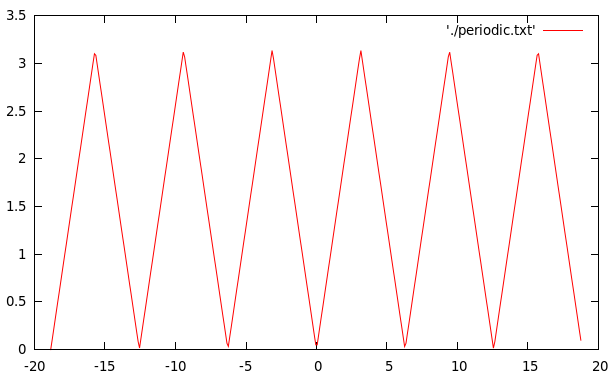# Triangular Wave (Periodic Function) – C Program

One might need to work with periodic functions in C for several reasons. Periodic functions are an important class of functions with numerous applications.

In this post I will show you how to define a particular periodic function in C, called the Triangular Wave.

But before that let me just give you a brief idea about dealing with periodic functions.

Periodic Functions are those that give the same value after a particular period.

Let’s say that there is a function f(x) which is periodic with a period of 2*T and is already defined in the interval [-T,T].

Then the function should have the same value at: f(x), f(x+2T), f(x+4T), ….

i.e. f(x)=f(x+2T)=f(x+4T)=……. since period=2*T.

But I said that the function is defined only in the interval [-T,T]. So how is the computer supposed to calculate it’s value at x>T?
That’s easy. Since the value of the function at f(x+2T) is simply f(x), therefore we can generalize that whenever x>T: then,
f(x)=f(x-2T).
Note: We have to keep taking x back by 2T i.e (x-2T) until it lies  within [-T,T] where the function is well-defined.

Similarly what about the value of function at x less than (-T) cause the function is not defined for values less than (-T)?
Again, this time we use:f(x)=f(x+2T). Note: We keep translating x forward by 2T  i.e (x+2*T) until it lies  within [-T,T] where the function is well-defined.

Using the above two arguments we can create a function which will make any given function defined within [-T,T] and with a period 2*T a periodic function.

The following program generates x and y-values for a Triangular Wave of period$2\pi$ defined by:

This means that the wave has an amplitude of$\pi$.
The calculated x and y-values are stored in a txt file called ‘periodic.txt’ and then plotted using Gnuplot.

### PROGRAM:

/************************************
*******PLOT A PERIODIC FUNCTION*****
************TRIANGULAR WAVE*********
***********************************/
#include<stdio.h>
#include<math.h>
/**Function definition for the Triangular Wave(Periodic Function)**/
double f(double x){
if(x>=0&&x<M_PI){
return x;
}
else if(x>=M_PI&&x<2*M_PI){
return 2*M_PI-x;
}
else if(x>=2*M_PI){
return f(x-2*M_PI);
}
else if(x<0){
return f(x+2*M_PI);
}
}
main(){
FILE *fp=NULL;
/*To write the data points to a txt file [periodic.txt]**/
fp=fopen("periodic.txt","w");
double x;
/**To plot in the rang of -6pi to 6pi **/
for (x=-6*M_PI;x<=6*M_PI;x=x+0.1){
fprintf(fp,"%lf\t%lf\n",x,f(x));
}
}


The above program will produce a file called ‘periodic.txt’ containing the data points for the Triangular Wave.
Now open, Gnuplot and plot it using the following command:
 plot 'periodic.txt' w l 
The result looks as shown.

### GNUPlot Output:[wpedon id="7041" align="center"]